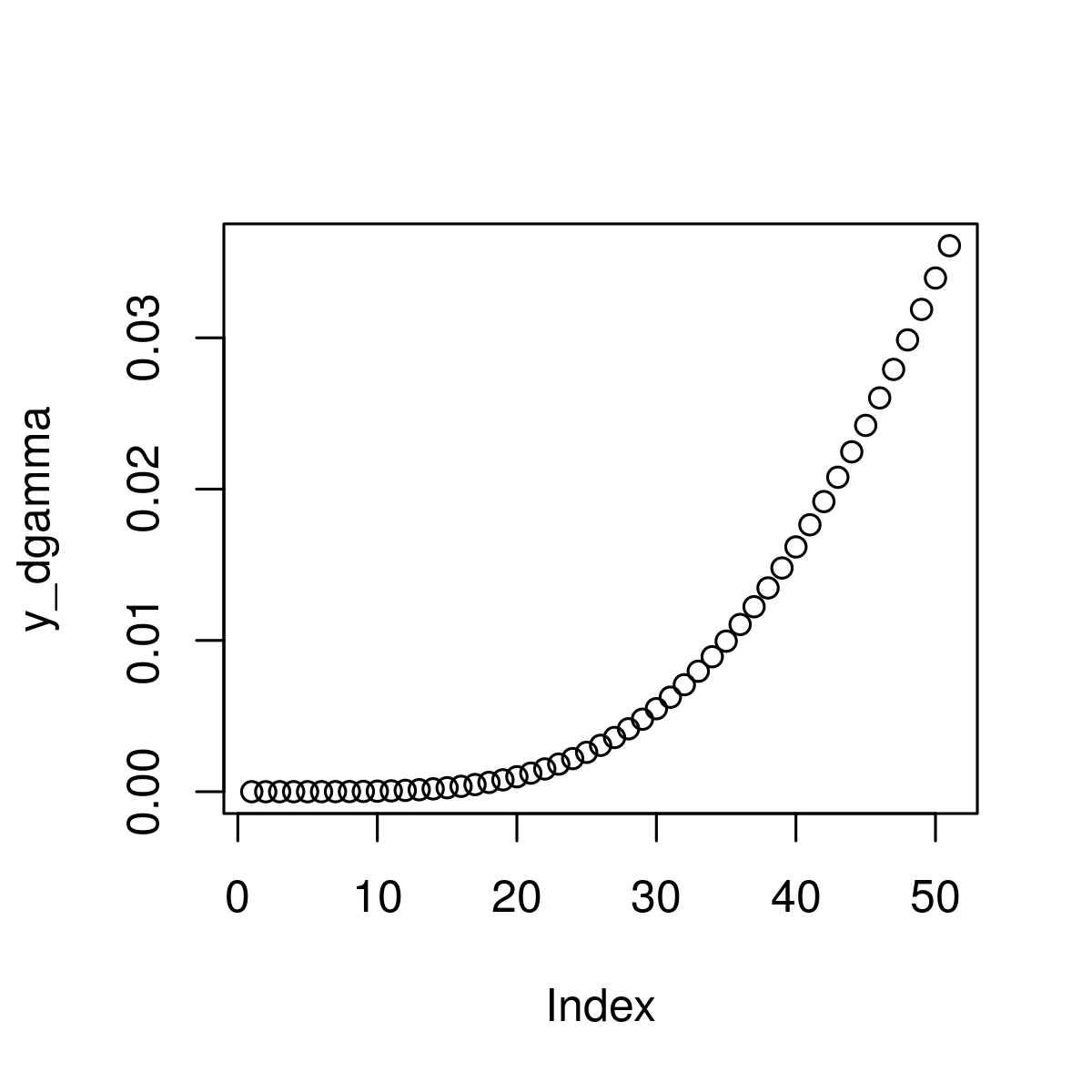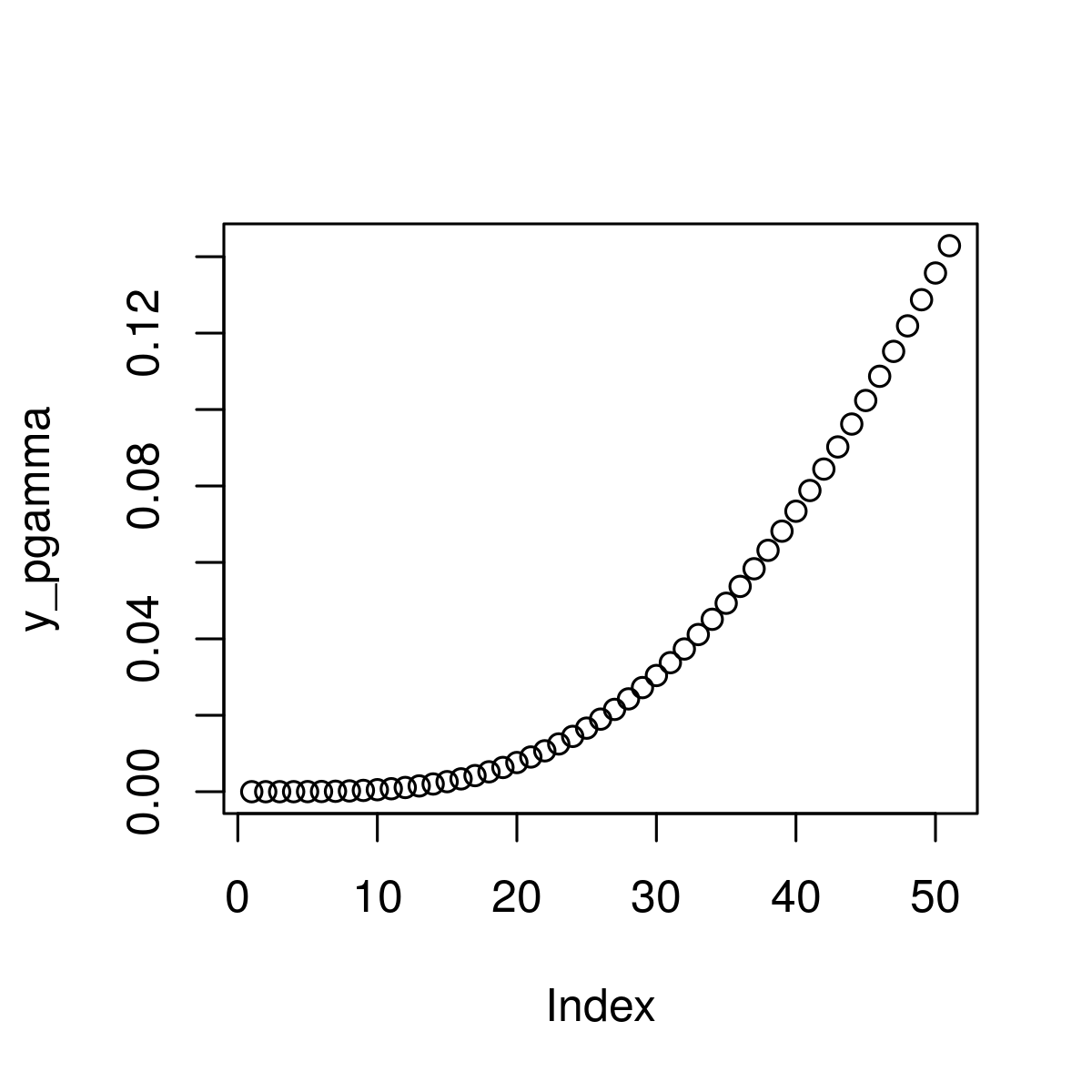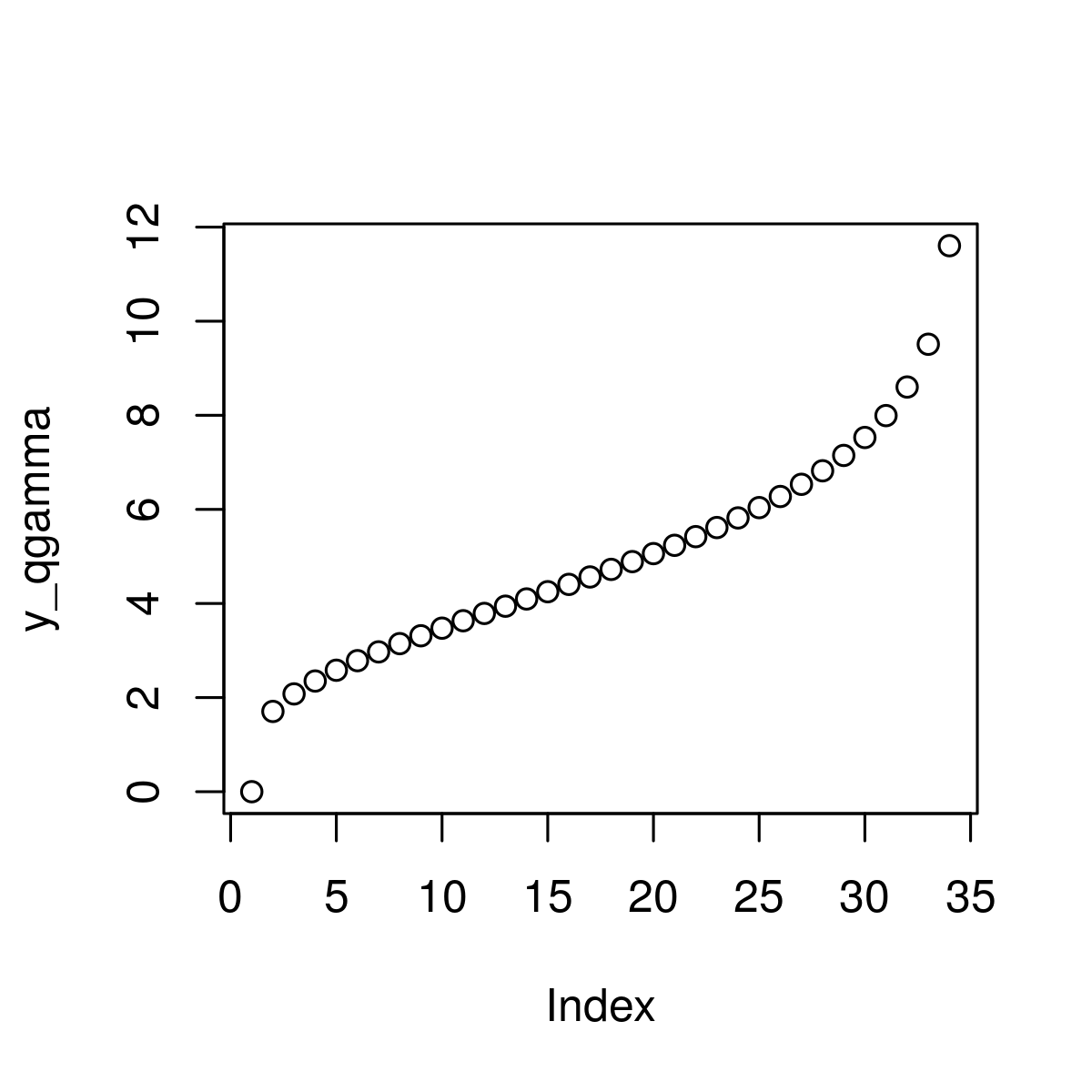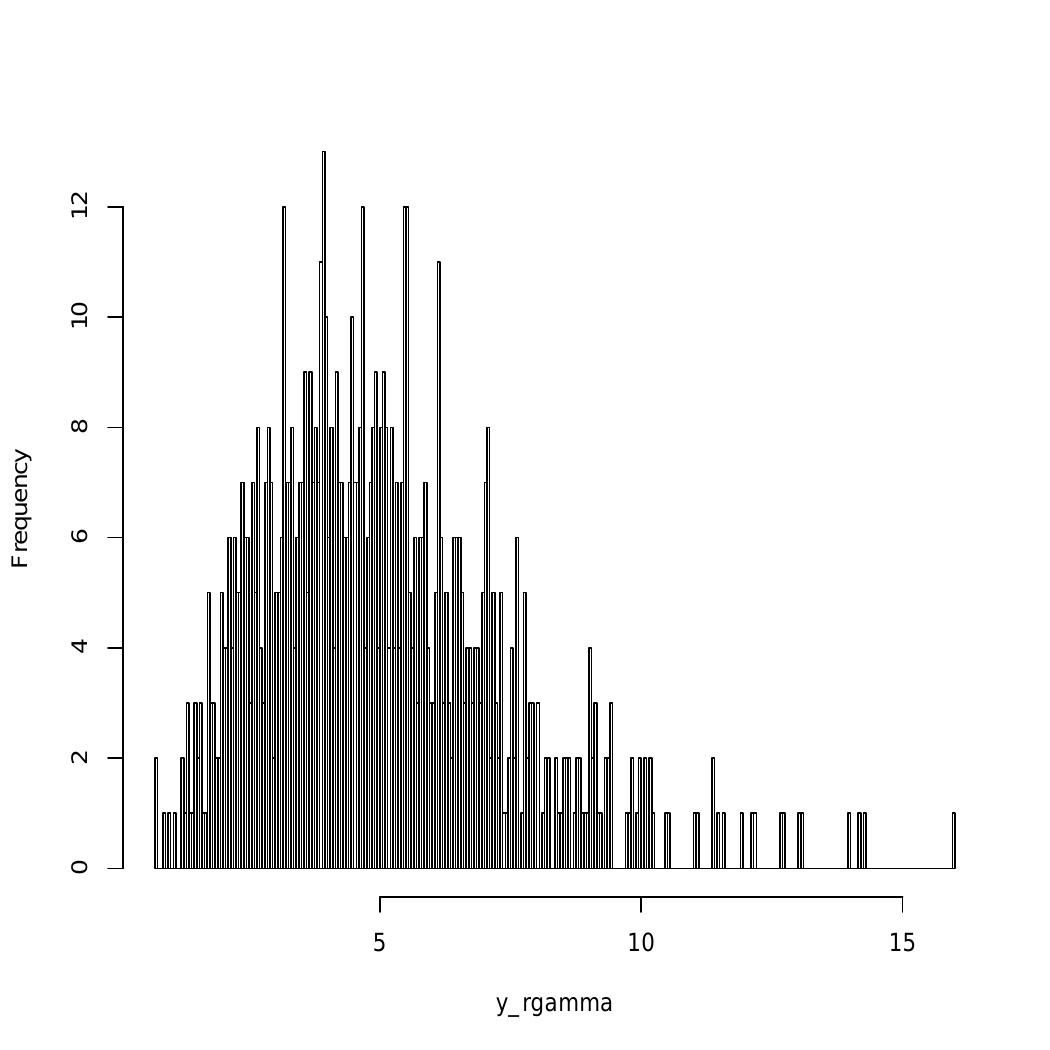# Gamma Distribution in R Programming – dgamma(), pgamma(), qgamma(), and rgamma() Functions

The Gamma distribution in R Language is defined as a two-parameter family of continuous probability distributions which is used in exponential distribution, Erlang distribution, and chi-squared distribution. This article is the implementation of functions of gamma distribution.

#### dgamma() Function

`dgamma()` function is used to create gamma density plot which is basically used due to exponential and normal distributions factors.

Syntax:
dgamma(x_dgamma, shape)

Parameters:
x_dgamma: defines gamma function
shape: gamma density of input values

Returns: Plot dgamma values

Example :

 `# R program to plot gamma distribution ` ` `  `# Specify x-values for gamma function ` `x_dgamma <- ``seq``(0, 2, by = 0.04)    ` ` `  `# Apply dgamma function ` `y_dgamma <- ``dgamma``(x_dgamma, shape = 6)  ` ` `  `# Plot dgamma values ` `plot``(y_dgamma) `

Output :#### pgamma() Function

`pgamma()` function is used in cumulative distribution function (CDF) of the gamma distribution.

Syntax:
pgamma(x_pgamma, shape)

Parameters:
x_pgamma: defines gamma function
shape: gamma density of input values

Returns: Plot pgamma values

Example:

 `# R program to plot gamma distribution ` ` `  `# Specify x-values for gamma function ` `x_pgamma <- ``seq``(0, 2, by = 0.04)    ` ` `  `# Apply pgamma function ` `y_pgamma <- ``pgamma``(x_pgamma, shape = 6)  ` ` `  `# Plot pgamma values ` `plot``(y_pgamma) `

Output:#### qgamma() Function

It is known as gamma quantile function of the gamma distribution and used to plot qgamma distribution.

Syntax:
qgamma(x_qgamma, shape)

Parameters:
x_qgamma: defines gamma function
shape: gamma density of input values

Returns: Plot qgamma values with gamma density

Example :

 `# R program to plot gamma distribution ` ` `  `# Specify x-values for gamma function ` `x_qgamma <- ``seq``(0, 1, by = 0.03)    ` ` `  `# Apply qgamma function ` `y_qgamma <- ``qgamma``(x_qgamma, shape = 6)  ` ` `  `# Plot qgamma values ` `plot``(y_qgamma) `

Output:#### rgamma() Function

This function is basically used for generating random number in gamma distribution.

Syntax:
rgamma(N, shape)

Parameters:
N: gamma distributed values
shape: gamma density of input values

Returns: Plot rgamma values with gamma density

Example :

 `# R program to plot gamma distribution ` ` `  `# Set seed for reproducibility ` `set.seed``(1200)  ` ` `  `# Specify sample size ` `N <- 800   ` ` `  `# Draw N gamma distributed values ` `y_rgamma <- ``rgamma``(N, shape = 5)  ` ` `  `# Print values to RStudio console ` `y_rgamma  ` ` `  `# Plot of randomly drawn gamma density ` `hist``(y_rgamma, breaks = 500, main = ``""``) `

Output:My Personal Notes arrow_drop_upCheck out this Author's contributed articles.

If you like GeeksforGeeks and would like to contribute, you can also write an article using contribute.geeksforgeeks.org or mail your article to contribute@geeksforgeeks.org. See your article appearing on the GeeksforGeeks main page and help other Geeks.

Please Improve this article if you find anything incorrect by clicking on the "Improve Article" button below.

Article Tags :

Be the First to upvote.

Please write to us at contribute@geeksforgeeks.org to report any issue with the above content.